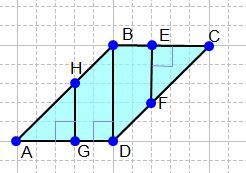• The learning process is entertaining and exciting
• The solved tasks turn into coordinates
• Along with your schoolmates and friends, go on an adventure, searching for hideouts
• E-mail:

# 2390.3. Distance between parallel straight lines.

Often, solving the tasks, where the area of the difficult geometric figures needs to be defined, it is profitable to divide them into simplier figures, for example, stright angled triangles, squares, rectangles. The area of all figures is possible to define, summing the areas of the separate figures.

The parallelogram ABCD is given. Which of the heights given (choose only one!) divide the parallelogram ABCD into simplier figures in a way, that it's area would be possible to calculate, knowing only the formulas of the triangle, the square and the rectangle?